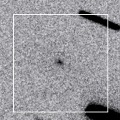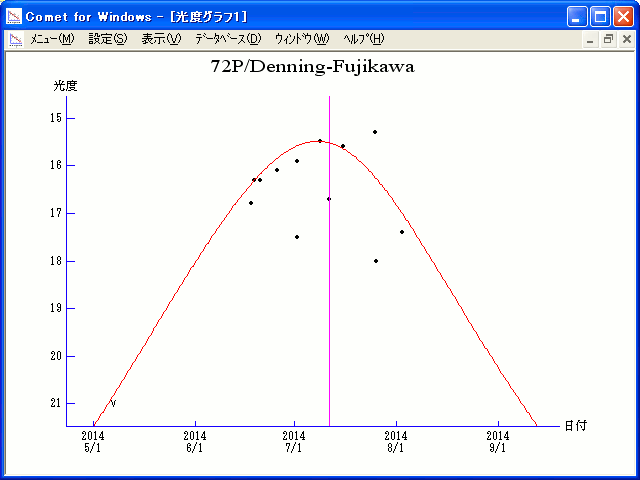# \$B%G%K%s%0(B-\$BF#@nWB@1(B

72P/Denning-Fujikawa (2014)###\$B%W%m%U%#!<%k(B

 \$B8!=PF|(B 2014\$BG/(B6\$B7n(B17\$BF|(B \$B8!=P8wEY(B 16.8\$BEy(B \$B8!=P Hidetaka Sato (Tokyo, Japan)

###\$B###\$B50F;MWAG(B

```Epoch 2014 July 2.0 TT = JDT 2456840.5
T 2014 July 11.62907 TT                                 MPC
q   0.7841523            (2000.0)            P               Q
n   0.10922578     Peri.  337.83984     +0.96763834     -0.23420748
a   4.3343032      Node    36.11656     +0.24876420     +0.82291922
e   0.8190823      Incl.    9.16963     +0.04233697     +0.51763965
P   9.02
From 26 observations 1881 Oct. 19-2014 June 18, mean residual 1".6.
Nongravitational parameters A1 = -0.06, A2 = +0.0240.
```

###\$B@1?^(B###\$B8wEYJQ2=(B

```        m1 = 17.5 + 5 log\$B&\$(B + 23.0 log r
```##### \$B50F;MWAG\$O(BM.P.E.C. 2014-M11\$B\$K7G:\\$5\$l\$?\$b\$N\$G\$9!#(B \$B@1?^\$O%9%F%i%J%S%2!<%?(B Ver.8 (\$B%"%9%H%m%"!<%D(B) \$B\$G:n@.\$7\$?\$b\$N\$G\$9!#(B \$B8wEY%0%i%U\$O(BComet for Windows\$B\$G:n@.\$7\$?\$b\$N\$G\$9!#(B